# Mapping Travel Times with malariaAtlas and Friction Surfaces

## Travel Time Tutorial

`## Packageslibrary(gdistance)library(abind)library(rje)library(ggplot2)library(malariaAtlas)## Plot defaultstheme_set(theme_minimal(base_size=14))`
`USA.shp <- malariaAtlas::getShp(ISO = "USA", admin_level = "admin1")analysis.shp <- USA.shp[USA.shp@data\$name=="Colorado",]plot(analysis.shp, main="Shape for Clipping")`
`friction <- malariaAtlas::getRaster(                      surface = "A global friction surface enumerating land-based travel speed for a nominal year 2015",                      shp = analysis.shp)malariaAtlas::autoplot_MAPraster(friction)`
`T <- gdistance::transition(friction, function(x) 1/mean(x), 8) T.GC <- gdistance::geoCorrection(T)                    `
`## Point locationspoint.locations <- read.csv(file = points.filename)names(point.locations) <- c("X_COORD", "Y_COORD", "name")# Keep only point coordinates within the shapefile boundscoordinates(point.locations) <- ~ X_COORD + Y_COORDproj4string(point.locations) <- proj4string(analysis.shp)overlap <- over(point.locations, analysis.shp)point.locations <- point.locations[!is.na(overlap\$gid),]`
`points <- as.matrix(point.locations@coords)`
`access.raster <- gdistance::accCost(T.GC, points)`
`p <- malariaAtlas::autoplot_MAPraster(access.raster,                         shp_df=analysis.shp, printed=F)full_plot <- p[] + geom_point(data=data.frame(point.locations@coords),                            aes(x=X_COORD, y=Y_COORD)) +scale_fill_gradientn(colors = rev(rje::cubeHelix(gamma=1.0,                                                  start=1.5,                                                  r=-1.0,                                                  hue=1.5,                                                  n=16)),                      name="Minutes \n of Travel") + ggtitle("Travel Time to Most Accessible Peak") +theme(axis.text=element_blank(),      panel.border=element_rect(fill=NA, color="white"))print(full_plot)`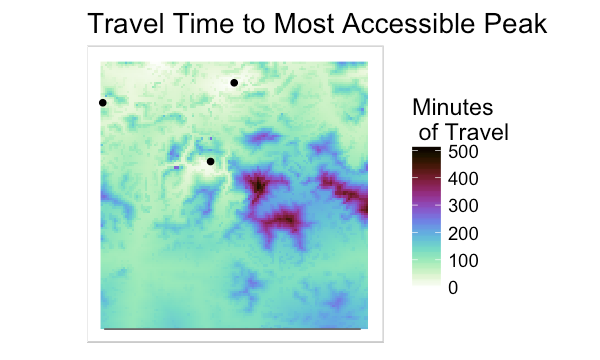Highlight of the south-central edge of the map, around Windom Peak.

by the author.

--

--

--

## More from Amelia Bertozzi-Villa

Modeler.

Love podcasts or audiobooks? Learn on the go with our new app.

## Child Trafficking in India - In depth analysis and visualisation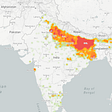## How to put error bars on your data: the easy way## Battle of neighbourhoods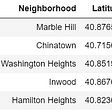## Find a location for opening a restaurant## Chapter 1 : Supervised Learning and Naive Bayes Classification — Part 2 (Coding)## The Poisson Deviance for Regression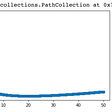## Let’s do some machine learning with R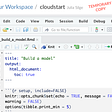Modeler.

## Why Data Analytics?## Table of installed packages in R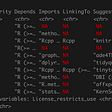## Cozy Collecting, Case Study: Getting a Decade’s Worth of Weather Data in R## Amazon Bestsellers — EDA & KMeans with R